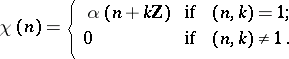# Character of a group

(diff) ← Older revision | Latest revision (diff) | Newer revision → (diff)

A homomorphism of the given group into some standard Abelian group. Usually,is taken to be either the multiplicative groupof a fieldor the subgroup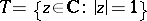of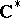. The concept of a character of a group was originally introduced for finite groupswith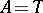(in this case every character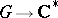takes values in).

The study of characters of groups reduces to the case of Abelian groups, since there is a natural isomorphism between the groupsand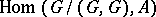, where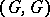is the commutator subgroup of. The characters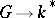form a linearly independent system in the space of all-valued functions on. A characterextends uniquely to a character of the group algebra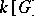. The charactersare one-dimensional linear representations ofover; the concept of a character of a representation of a group coincides in the one-dimensional case with the concept of a character of a group. Sometimes characters of a group are understood to mean characters of any of its finite-dimensional representations (and even to mean the representations themselves).

A character of a topological groupis a continuous homomorphism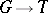. Ifis a locally compact Abelian group, then its characters separate points, that is, for any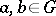,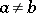, there exists a charactersuch that. For Hausdorff Abelian groupsthis assertion is not true, in general (see ). A character of an algebraic group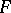over an algebraically closed fieldis a rational homomorphism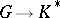.

In number theory an important role is played by the characters of the multiplicative groupof the residue ringmodulo, which correspond one-to-one to Dirichlet characters modulo: To a character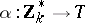there corresponds the Dirichlet charactergiven by the formula